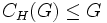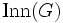# Characteristically simple and non-abelian implies monolith in automorphism group

## Statement

If$G$ is a Characteristically simple group (?) that is non-abelian, then$G$ is a Monolith (?) in its Automorphism group (?). In particular, the automorphism group of$G$ is a Monolithic group (?).

In particular, this shows that the automorphism group of any Simple non-abelian group (?) is a complete group.

## Facts used

1. Center is characteristic
2. Centerless implies NSCFN in automorphism group
3. Characteristically simple and NSCFN implies monolith: If$G$ is a characteristically simple subgroup of a group$H$, such that$G$ is normal in$H$,$C_H(G) \le G$, and every automorphism of$G$ extends to an inner automorphism of$H$,$G$ is a the unique nontrivial normal subgroup of$H$ contained in every nontrivial normal subgroup of$H$.

## Proof

Given: A characteristically simple non-abelian group$G$, with automorphism group$\operatorname{Aut}(G)$.

To prove:$\operatorname{Aut}(G)$ is complete: it is centerless and every automorphism of it is inner.

Proof:

1. (Given data used:$G$ is characteristically simple non-abelian; Facts used: fact (1)):$G$ is centerless: By fact (1), the center of$G$ is characteristic in$G$. Since$G$ is non-abelian, the center of$G$ is not equal to$G$. Since$G$ is characteristically simple, this forces the center of$G$ to be trivial.
2. The natural mapping from$G$ to$\operatorname{Aut}(G)$ given by the conjugation action is injective, identifying$G$ with its image$\operatorname{Inn}(G)$: This follows from the fact that$G$ is centerless, so the kernel of the map is trivial.
3. (Facts used: fact (2)):$G$ is normal, self-centralizing and fully normalized in$\operatorname{Aut}(G)$: This follows from fact (2), and the fact that$G$ is centerless.
4. (Given data used:$G$ is characteristically simple; Facts used: fact (3)): By fact (3) and the conclusion of the previous step (step (3)), and the given datum that$G$ is characteristically simple, we conclude that$G$ is a monolith in$\operatorname{Aut}(G)$: it is the unique nontrivial normal subgroup of$\operatorname{Aut}(G)$ that is contained in every nontrivial normal subgroup of$\operatorname{Aut}(G)$.